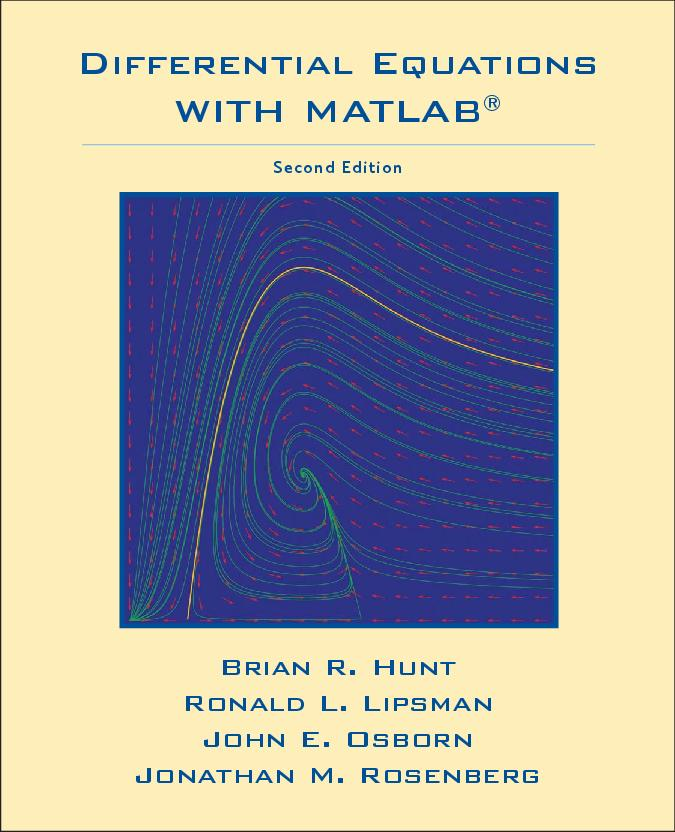Home
Project Description
Authors

Differential Equations
with Matlab

Differential Equations
with Mathematica

Differential Equations
with Maple# Differential Equations with MATLAB, 2nd Edition

## Table of Contents

Preface iii
1 Introduction 1
1.1 Guiding Philosophy 1
1.2 Student's Guide 3
1.3 Instructor's Guide 4
1.4 A Word About Software Versions 6
2 Getting Started with MATLAB 7
2.1 Platforms and Versions 7
2.2 Installation 8
2.3 Starting MATLAB 8
2.4 Typing in the Command Window 9
2.5 Online Help 9
2.6 MATLAB Windows 11
2.7 Ending a Session 12
3 Doing Mathematics with MATLAB 13
3.1 Arithmetic 13
3.2 Recovering from Problems 14
3.3 Symbolic Computation 15
3.4 Vectors 17
3.5 Functions 18
3.6 Managing Variables 20
3.7 Solving Equations 21
3.8 Graphics 23
3.9 Calculus 29
3.10 Some Tips and Reminders 30
4 Using M-files and M-books 31
4.1 The MATLAB Desktop 31
4.2 M-files 34
4.3 Loops 38
4.4 Presenting Your Results 38
4.5 Debugging Your M-files 45
Problem Set A: Practice with MATLAB 47
5 Solutions of Differential Equations 51
5.1 Finding Symbolic Solutions 51
5.2 Existence and Uniqueness 54
5.3 Stability of Differential Equations 55
5.4 Different Types of Symbolic Solutions 59
6 A Qualitative Approach to Differential Equations 65
6.1 Direction Field for a First Order Linear Equation 65
6.2 Direction Field for a Non-Linear Equation 68
6.3 Autonomous Equations 69
Problem Set B: First Order Equations 75
7 Numerical Methods 87
7.1 Numerical Solutions Using MATLAB 88
7.2 Some Numerical Methods 91
7.3 Controlling the Error in ode45 98
7.4 Reliability of Numerical Methods 99
8 Features of MATLAB 103
8.1 Data Classes 103
8.2 Functions and Expressions 106
8.3 More about M-files 107
8.4 Matrices 109
8.5 Graphics 111
8.6 Features of MATLAB 's Numerical ODE Solvers 114
8.7 Troubleshooting 119
9 Using Simulink 121
9.1 Constructing and Running a Simulink Model 121
9.2 Output to the Workspace and How Simulink Works 127
Problem Set C: Numerical Solutions 131
10 Solving and Analyzing Second Order Linear Equations 139
10.1 Second Order Equations with MATLAB 141
10.2 Second Order Equations with Simulink 145
10.3 Comparison Methods 147
10.4 A Geometric Method 150
Problem Set D: Second Order Equations 157
11 Series Solutions 171
11.1 Series Solutions 172
11.2 Singular Points 174
11.3 Function M-files for Series Solutions 177
11.4 Series Solutions Using maple 180
12 Laplace Transforms 183
12.1 Differential Equations and Laplace Transforms 185
12.2 Discontinuous Functions 188
12.3 Differential Equations with Discontinuous Forcing 190
Problem Set E: Series Solutions and Laplace Transforms 193
13 Higher Order Equations and Systems of First Order Equations 207
13.1 Higher Order Linear Equations 208
13.2 Systems of First Order Equations 209
13.3 Phase Portraits 216
14 Qualitative Theory for Systems of Differential Equations 223
Problem Set F: Systems of Differential Equations 231
Glossary 247
Sample Solutions 259
Index 285